Next: Laboratory Schiff Moment of Up: Calculating the Schiff moment Previous: Calculating the Schiff moment

## Odd-Nuclei and Schiff Moments in Mean-Field Approximation

Fully self-consistent calculations in odd-nuclei are possible but seldom performed. For many physical observables it is enough to neglect correlations between the odd particle and the core, which amounts to dropping a valence particle into the field generated by that core. Other quantities, however, are sensitive to the interaction between the last particle and the core. The interaction can change the deformation and pairing strength, and produce various kinds of core polarization. In fact, a self-consistent odd-calculation is equivalent to first performing the calculation in the even-even nucleus with one less neutron, then placing the last nucleon in the first empty orbit and treating its polarizing effect on the core in the RPA [Blaizot and Ripka(1986)]. Fully self-consistent calculations take all kinds of polarization into account simultaneously. For us, the spin polarization is of particular interest.

To proceed here we use the public-domain Skyrme-Hartree-Fock code HFODD (v2.04b) [Dobaczewski and Dudek(1997),Dobaczewski and Dudek(2000),Olbratowski et al.()Olbratowski, Dobaczewski, and Dudek], which allows the mean-field and the associated Slater determinant to simultaneously break invariance under rotation, reflection through three planes, and time reversal (the code we used in the last section does not). Breaking the first is necessary to describe a deformed nucleus. Breaking all three reflections (and not only parity) is necessary to represent axial octupole deformation with the spin aligned along the symmetry axis. Breaking the last induces spin polarization in the core, which because of Kramers degeneracy cannot otherwise occur. Incorporating spin polarization is important because it has the potential to significantly alter the matrix element of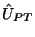in Eq. (8) from its value in the particle-rotor model, where the spin is carried entirely by one valence particle. The code HFODD cannot yet treat pairing when it allows T to be broken, but pairing in T-odd channels is poorly understood. No existing codes can do more than HFODD in odd-octupole-deformed nuclei.

As above, we use the Skyrme interactions SIII, SkM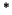, SLy4, and SkO'. The reason SkO' is our favorite has to do with the part of the energy functional composed of T-odd spin densities (which, following common practice, we refer to as the T-odd functional", even though the entire functional must be even under T). The T-odd functional plays no role in the mean-field ground states of even nuclei, but can be important in any state with nonzero angular momentum. Of the forces above, only SkO' has been seriously investigated in T-odd channels. In Ref. [Bender et al.(2002)Bender, Dobaczewski, Engel, and Nazarewicz], the T-odd part of the functional was adjusted to reproduce Gamow-Teller resonances, resulting in an effective Landau parameter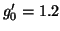. In the isoscalar channel, the force was adjusted to reproduce the commonly used value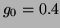[Osterfeld(1992)]. Although there are not enough data to constrain other relevant parameters in the functional, and although a very recent calculation starting from a realistic nucleon-nucleon interaction [Zuo et al.(2003)Zuo, Shen, and Lombardo], while confirming the value, finds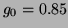, SkO' is clearly the best available Skyrme interaction for describing spin-spin interactions. The corresponding T-odd terms in the functional are precisely those that will polarize the spin in the core. There are other terms that could be added to the standard Skyrme interaction and do the same thing -- the tensor force for example -- but they are almost never used and their effects still need to be investigated.

The parameters of the other three forces SIII, SkM, and SLy4 were adjusted entirely to ground-state properties in even-even nuclei, and so the Landau parameters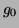and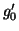were not fit. Here we set them to zero by treating the T-odd and T-even terms in the Skyrme functional independently, as described in Ref. [Bender et al.(2002)Bender, Dobaczewski, Engel, and Nazarewicz]. Only orbital terms, which are fixed by gauge invariance [Dobaczewski and Dudek(1995)] (a generalization of Galilean invariance), appear in the T-odd parts of these forces.

We rely on the crudest forms of projection. For parity, that means none at all, and for angular momentum it means inserting the rigid-rotor factor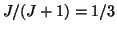in front of the intrinsic Schiff moment, as described above. In other words, we use Eq. (6), with the intrinsic state taken to be the Hartree-Fock ground state produced by HFODD. Just as in the particle-rotor model, the intrinsic Schiff moment is given by the classical expression Eq. (1), but with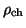equal to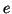times the Hartree-Fock ground-state proton density. As already mentioned, the Hartree-Fock approximation allows us, by summing over occupied orbits, to write the intrinsic matrix element of the two-body potential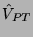as the expectation value of an effective one-body operator. Because we now have a microscopic version of the core", this effective potential is more complicated than in Eq. (8), and it now acts on all the nucleons: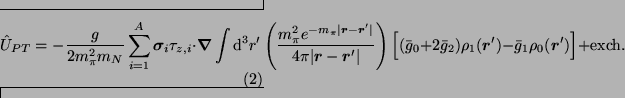Here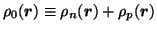and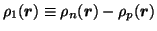are the isoscalar and isovector densities. The piece coming from exchange terms in the original two-body interactionis nonlocal, just as in the usual Hartree-Fock mean field, and we have not written it explicitly here (though we do below).

The code HFODD at present cannot evaluate the expectation value of a folded potential like that above, which is due to the finite pion range. Nevertheless, even in the zero-range approximation we can avoid several of the assumptions -- proportionality of neutron and proton densities, negligibility of exchange terms, and absence of core spin -- leading to the extremely simplified potential in Eq. (8). The zero-range approximation is equivalent to assuming the pion is very heavy, so that the term involving the pion mass in Eq. (9) becomes a delta function. Under that assumption, but none others, the exchange terms become local andtakes the form: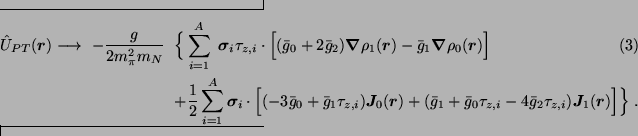Here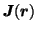is the spin-orbit" current, defined, e.g., in Ref. [Bender et al.(2002)Bender, Dobaczewski, Engel, and Nazarewicz] and references therein, and the subscripts 0 and 1 refer to isoscalar and isovector combinations as they do for the density. The terms inthat contain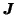are the exchange terms omitted above. We will evaluate them, but argue later that their effects are probably small when the finite range is restored. The terms containing the density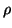all come from the direct part of. We do not simplify things further to obtain something like Eq. (8) because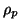is not really proportional to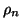and the core nucleons do carry some spin. We will manage nevertheless, to compare our results with those of Ref. [Spevak et al.(1997)Spevak, Auerbach, and Flambaum]. We will also estimate the effect of a finite pion range on the direct terms, though our inability to do so more precisely at present is the most significant shortcoming of this work.

HFODD works by diagonalizing the interaction in the eigenbasis of an optimal anisotropic three-dimensional harmonic oscillator. For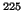Ra, algorithms developed in Ref. [Dobaczewski and Dudek(1997)] give oscillator frequencies of=7.0625 and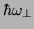=8.6765 MeV in the directions parallel and perpendicular to the elongation axis. The matrix element ofconverges only slowly as we increase the number of levels in the basis. When the interaction polarizes the core, it takes 2500 or more single-particle basis states to get convergence. The basis then contains up to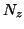=26 and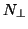=21 oscillator quanta.Next: Laboratory Schiff Moment of Up: Calculating the Schiff moment Previous: Calculating the Schiff moment
Jacek Dobaczewski 2003-04-24